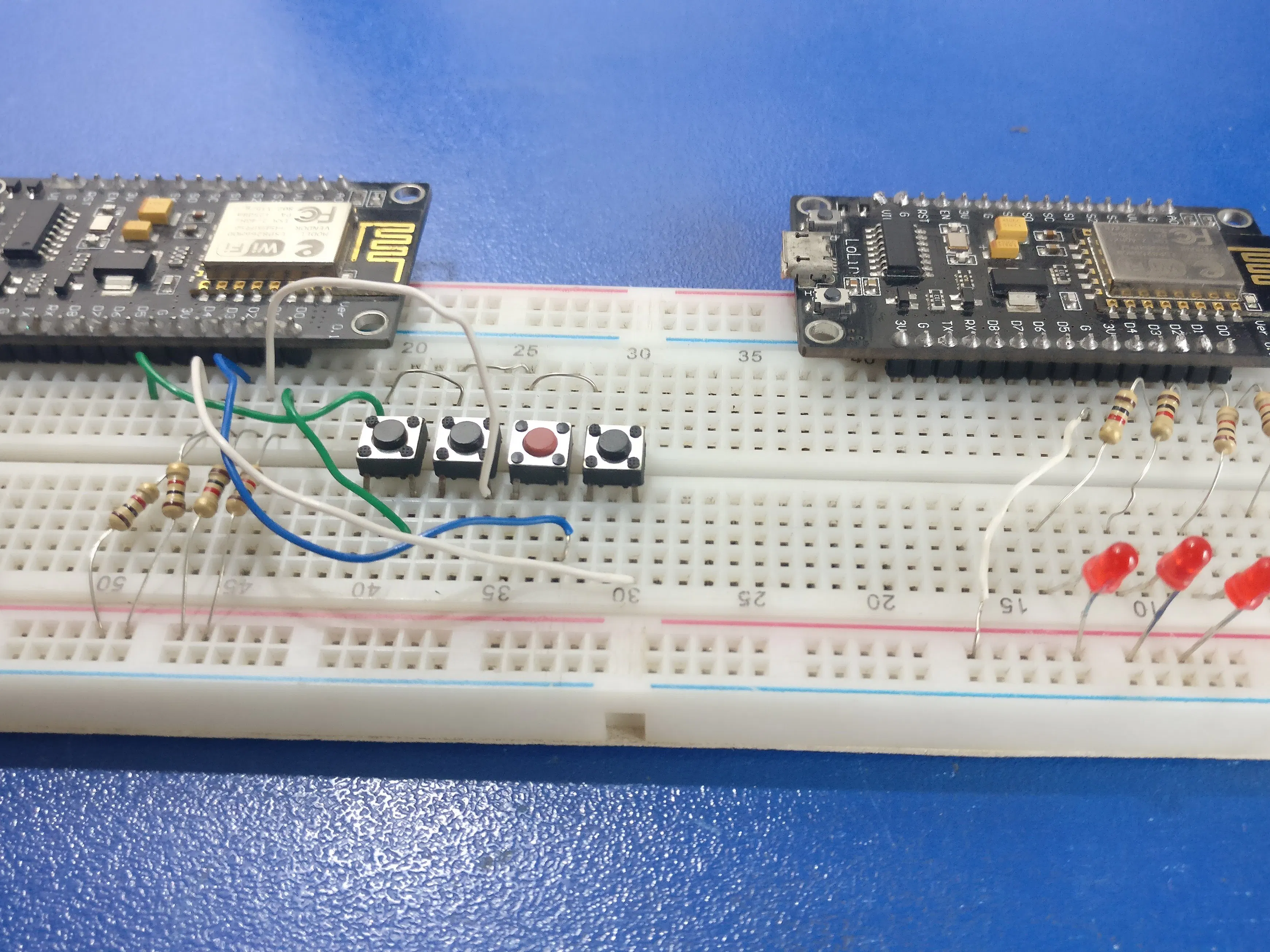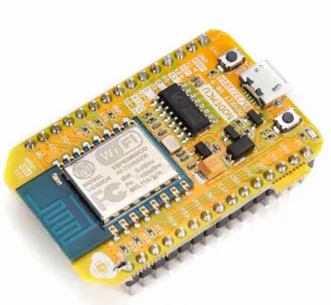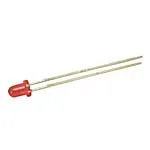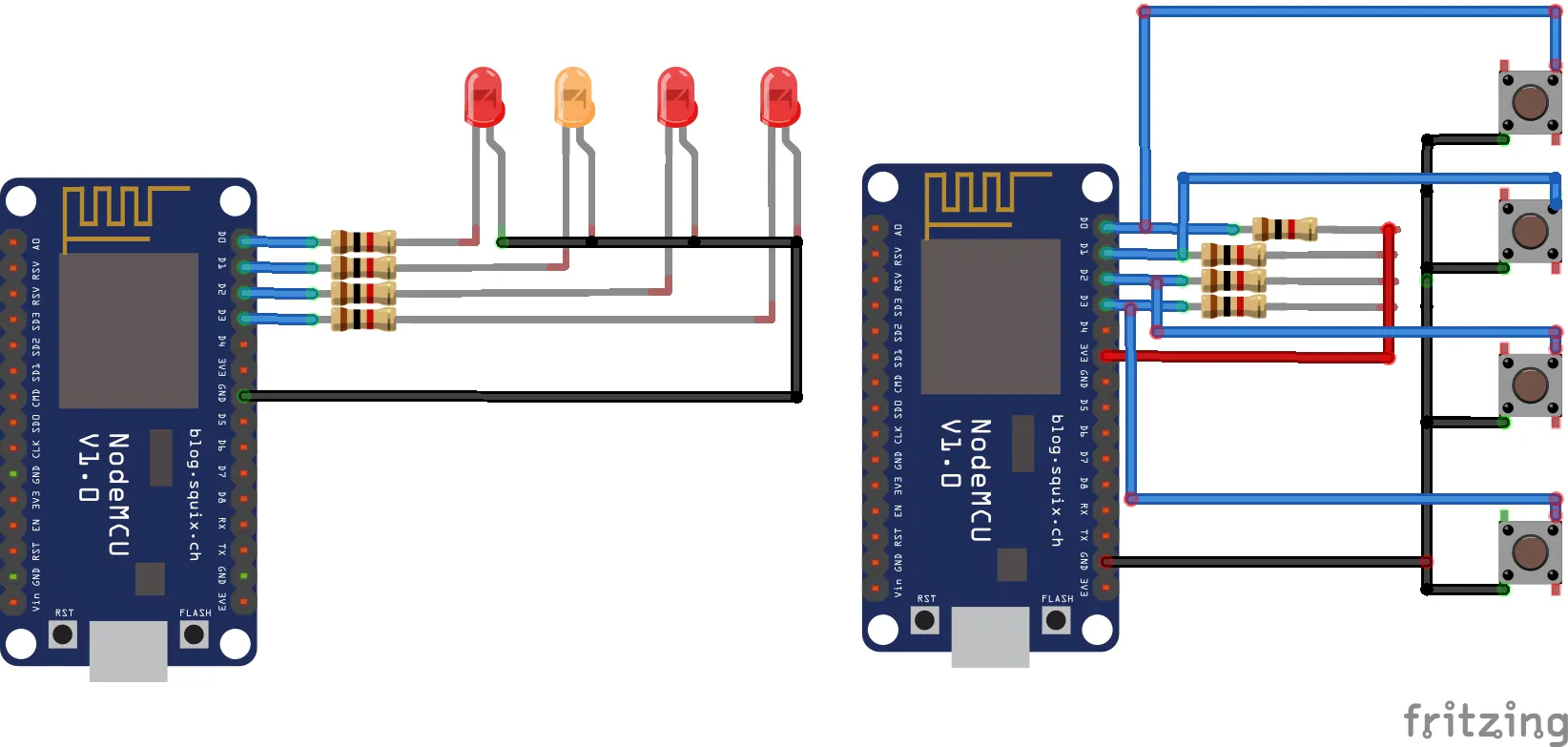# ESP8266 to ESP8266 Direct Communication

This tutorial will help you to replace other transceiver modules for any other project that includes wireless communication.

IntermediateFull instructions provided1 hour54,034## Things used in this project

### Hardware componentsNodeMCU ESP8266 Breakout Board
×1SparkFun Pushbutton switch 12mm
×13 mm LED: Red
×1Resistor 1k ohm
×1

### Software apps and online servicesArduino IDE

## Schematics

### Circuit Diagram

Buttons at Server side, LEDs at client side## Code

### Server

C/C++
```//hammadiqbal12@gmail.com

#include <ESP8266WiFi.h>

#define btn0 16
#define btn1 5
#define btn2 4
#define btn3 0

const char *ssid = "ssid";

int sensorValue0 = 0;       //sensor value, I'm usingg 0/1 button state
int sensorValue1 = 0;
int sensorValue2 = 0;
int sensorValue3 = 0;

void setup() {
Serial.begin(115200);
delay(10);

pinMode(btn0, INPUT);
pinMode(btn1, INPUT);
pinMode(btn2, INPUT);
pinMode(btn3, INPUT);

// set the ESP8266 to be a WiFi-client
WiFi.mode(WIFI_STA);

while (WiFi.status() != WL_CONNECTED) {
delay(500);
}

}

void loop() {
if(digitalRead(btn0) == LOW) sensorValue0 = 1;
if(digitalRead(btn1) == LOW) sensorValue1 = 1;
if(digitalRead(btn2) == LOW) sensorValue2 = 1;
if(digitalRead(btn3) == LOW) sensorValue3 = 1;

if(digitalRead(btn0) == HIGH) sensorValue0 = 0;
if(digitalRead(btn1) == HIGH) sensorValue1 = 0;
if(digitalRead(btn2) == HIGH) sensorValue2 = 0;
if(digitalRead(btn3) == HIGH) sensorValue3 = 0;

// Use WiFiClient class to create TCP connections
WiFiClient client;
const char * host = "192.168.4.1";            //default IP address
const int httpPort = 80;

if (!client.connect(host, httpPort)) {
Serial.println("connection failed");
return;
}

// We now create a URI for the request. Something like /data/?sensor_reading=123
String url = "/data/";

url.replace("sensor0_value", String(sensorValue0));
url.replace("sensor1_value", String(sensorValue1));
url.replace("sensor2_value", String(sensorValue2));
url.replace("sensor3_value", String(sensorValue3));

// This will send the request to the server
client.print(String("GET ") + url + " HTTP/1.1\r\n" +
"Host: " + host + "\r\n" +
"Connection: close\r\n\r\n");
unsigned long timeout = millis();
while (client.available() == 0) {
if (millis() - timeout > 5000) {
Serial.println(">>> Client Timeout !");
client.stop();
return;
}
}
}
```

### Client

C/C++
```//hammadiqbal12@gmail.com

#include <ESP8266WiFi.h>
#include <ESP8266WebServer.h>
#include <ArduinoJson.h>

#define led0 16               //D0
#define led1 5                //D1
#define led2 4                //D2
#define led3 0                //D3

DynamicJsonBuffer jsonBuffer;

const char *ssid      = "ssid";

int sensorValue0 = 0;
int sensorValue1 = 0;
int sensorValue2 = 0;
int sensorValue3 = 0;
String sensor_values;

ESP8266WebServer server(80);

void handleSentVar() {

{
Serial.println(sensor_values);
}
JsonObject& root = jsonBuffer.parseObject(sensor_values);
//  if (!root.success()) {
//    Serial.println("parseObject() failed");
//    return;
//  }
//  if (root.success())
//  {

//  }

Serial.println(sensorValue0);
Serial.println(sensorValue1);
Serial.println(sensorValue2);
Serial.println(sensorValue3);

toggle_leds();

}

void setup() {
Serial.begin(9600);

pinMode(led0, OUTPUT);
pinMode(led1, OUTPUT);
pinMode(led2, OUTPUT);
pinMode(led3, OUTPUT);

//toggle_leds();                 //turn off all leds as all the sensor values are zero

server.on("/data/", HTTP_GET, handleSentVar); // when the server receives a request with /data/ in the string then run the handleSentVar function
server.begin();
}

void loop() {
server.handleClient();
}

void toggle_leds()
{
if (sensorValue0 == 0)  digitalWrite(led0, LOW);
if (sensorValue1 == 0)  digitalWrite(led1, LOW);
if (sensorValue2 == 0)  digitalWrite(led2, LOW);
if (sensorValue3 == 0)  digitalWrite(led3, LOW);

if (sensorValue0 == 1)  digitalWrite(led0, HIGH);
if (sensorValue1 == 1)  digitalWrite(led1, HIGH);
if (sensorValue2 == 1)  digitalWrite(led2, HIGH);
if (sensorValue3 == 1)  digitalWrite(led3, HIGH);
}
```

### Json Library

C/C++
`No preview (download only).`